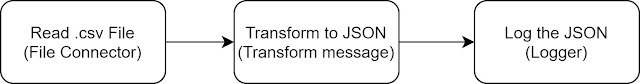## Posts

Showing posts from August, 2021

### Tips and Tricks for handling numbers in BPEL or OIC

While integrating with multiple systems and moving data from one system to the other, we generally come across scenarios, wherein we need to change the format of the number or perform operations like floor, ceil, round, divide, multiply, add, etc. on numbers. We will be going through various mathematical functions available in XSLT 1.0, which would be helpful in such scenarios, and also some generic errors which we encounter usually. - Mathematical Operations Divide Multiply Add Subtract Square-root Round Floor Ceil Advanced Number formatting - Common Errors Mathematical Operations 1. Divide To divide 2 numbers, we can use the  div operator to divide two numbers. Syntax:        \$num1 div \$num2 Example:        Let us assume we want to divide 120 by 7. XSL: <divide><xsl:value-of select="120 div 7"/></divide> Output <divide>17.14285714285714285714</divide&g

### Reading .csv files with and without headers using different delimiters in Mule 4